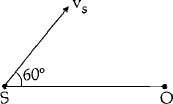## Filters

Sort by :
Clear All
Q
Medical
101 Views   |

If vectorsandare functions or time, then the value of t at which they are orthogonal to each other is :

• Option 1)• Option 2)• Option 3)• Option 4)As we learnt in Composition of two SHM in perpendicular direction -     - wherein Resultant equation     When they are orthogonal,  Correct option is 2.       Option 1) This option is incorrect  Option 2) This option is correct  Option 3) This option is incorrect  Option 4) This option is incorrect
Medical
95 Views   |

A particle is executing a simple harmonic motion. Its maximum acceleration isand maximum velocity is. Then, its time period of vibration will be :

• Option 1)• Option 2)• Option 3)• Option 4)As we learnt in Equation of S.H.M. -   - wherein          (Maximum acceleration)     (Maximum velocity) Correct option is 3. Option 1) This option is incorrect. Option 2) This option is incorrect. Option 3) This option is correct. Option 4) This option is incorrect.
Medical
285 Views   |

A source of sound S emitting waves of frequency 100 Hz and an observer O are located at some distance from each other. The source is moving with a speed of 19.4 ms-1 at an angle of 60° with the source observer line as shown in the figure. The observer is at rest. The apparent frequency observed by the observer (velocity of sound in air 330 ms-1), is:• Option 1)

103 Hz

• Option 2)

106 Hz

• Option 3)

97 Hz

• Option 4)

100 Hz

As discussed in frequency of sound when source is moving towards the observer and observer is moving away from source - - wherein Speed of sound Speed of observer speed of source Original frequency apparent frequency   From Doppler's effect,              Option 1) 103 Hz This option is correct Option 2) 106 Hz This option is incorrect Option 3) 97 Hz This option is incorrect Option...
Medical
107 Views   |

A string is stretched between fixed points separated by 75.0 cm. It is observed to have resonant frequencies of 420 Hz and 315 Hz. There are no other resonant frequencies between these two. The lowest resonant frequency for this string is:

• Option 1)

205 Hz

• Option 2)

10.5 Hz

• Option 3)

105 Hz

• Option 4)

155 Hz

As we discussed in Fundamental frequency with end correction -     (one end open)     (Both end open) e = end correction -   In a stretched string, all multiples of frequencies can be obtained, i.e., If fundamental frequency is , then higher frequencies will be  According to question,  Option 1) 205 Hz This option is incorrect. Option 2) 10.5 Hz This option is incorrect. Option 3) 105...
Exams
Articles
Questions